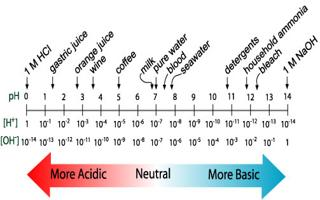Chemistry

# Acidic Basic

In an acidic solution, the concentration of H+ (aq) is greater than that of OH+ (aq). After dissolving substances in water, one can alter the concentrations of H+ (aq) and OH-(aq).

In acidic basic solution, the concentration of OH- (aq) is greater than that of H+ (aq). In a neutral solution, the concentrations of H+ (aq) and OH (aq) are equal, as they are in pure water. Using the ion-product expression

KW = [H+] [OH]At 25°C, KW = 1.0 x 1014 so

• In an acidic solution, [H+] > 1.0 x 10-7 M
• In a neutral solution, [H+] = 1.0 x 10-7 M
• In a basic solution, [H+] < 1.0 x 10-7 M